# Some Examples of Units in Number Fields

The classical Pell's equation is, given square-free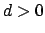, to find all positive integer solutions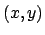to the equation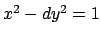. Note that if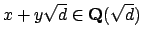, then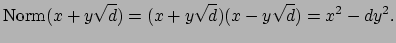The solutions to Pell's equation thus form a finite-index subgroup of the group of units in the ring of integers of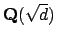. Dirichlet's unit theorem implies that for any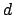the solutions to Pell's equation form an infinite cyclic group, a fact that takes substantial work to prove using only elementary number theory (for example, using continued fractions).

We first solve the Pell equation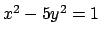by finding the units of a field using (we will likely discuss algorithms for computing unit groups later in the course...).

> R<x> := PolynomialRing(RationalField());
> K<a> := NumberField(x^2-5);
> G, phi := UnitGroup(K);
> G;
Abelian Group isomorphic to Z/2 + Z
Defined on 2 generators
Relations:
2*G.1 = 0
> K!phi(G.1);
-1
> u := K!phi(G.2); u;
1/2*(a + 1)
> u^2;
1/2*(a + 3)
> u^3;
a + 2
> Norm(u);
-1
> Norm(u^3);
-1
> Norm(u^6);
1
> fund := u^6;
> fund;
4*a + 9
> 9^2 - 5*4^2;
1
> fund^2;
72*a + 161
> fund^3;
1292*a + 2889
> fund^4;
23184*a + 51841
> fund^5;
416020*a + 930249


I think in practice for solving Pell's equation it's best to use the ideas in the paper [Len02]. A review of this paper says: This wonderful article begins with history and some elementary facts and proceeds to greater and greater depth about the existence of solutions to Pell equations and then later the algorithmic issues of finding those solutions. The cattle problem is discussed, as are modern smooth number methods for solving Pell equations and the algorithmic issues of representing very large solutions in a reasonable way.'' You can get the paper freely online from the Notices web page.

The simplest solutions to Pell's equation can be huge, even whenis quite small. Read Lenstra's paper for some awesome examples from antiquity.

K<a> := NumberField(x^2-NextPrime(10^7));
> G, phi := UnitGroup(K);
> K!phi(G.2);
1635802598803463282255922381210946254991426776931429155067472530\
003400641003657678728904388162492712664239981750303094365756\
106316392723776016806037958837914778176119741840754457028237\
899759459100428895693238165048098039*a +
517286692885814967470170672368346798303629034373575202975075\
605058714958080893991274427903448098643836512878351227856269\
086856679078304979321047765031073345259902622712059164969008\
6336036036403311756634562204182936222240930


The Signature command returns the number of real and complex conjugate embeddings of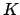into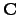. The command UnitGroup, which we used above, returns the unit group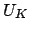as an abstract abelian group and a homomorphism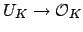. Note that we have to bang (!) intoto get the units as elements of.

First we consider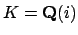.

> R<x> := PolynomialRing(RationalField());
> K<a> := NumberField(x^2+1);
> Signature(K);
0 1    // r=0, s=1
> G,phi := UnitGroup(K);
> G;
Abelian Group isomorphic to Z/4
Defined on 1 generator
Relations:
4*G.1 = 0
> K!phi(G.1);
-a


Next we consider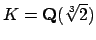.

> K<a> := NumberField(x^3-2);
> Signature(K);
1 1
> G,phi := UnitGroup(K);
> G;
Abelian Group isomorphic to Z/2 + Z
Defined on 2 generators
Relations:
2*G.1 = 0
> K!phi(G.2);
-a + 1

The Conjugates command returns the sequence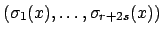of all embeddings of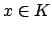into. The Logs command returns the sequence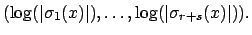Continuing the above example, we have
> Conjugates(K!phi(G.2));
[ -0.25992104989487316476721060727822835057025146470099999999995,
1.6299605249474365823836053036391141752851257323513843923104 -
1.09112363597172140356007261418980888132587333874018547370560*i,
1.6299605249474365823836053036391141752851257323513843923104 +
1.09112363597172140356007261418980888132587333874018547370560*i ]
> Logs(K!phi(G.2));   // image of infinite order unit -- generates a lattice
[ -1.34737734832938410091818789144565304628306227332099999999989\
, 0.6736886741646920504590939457228265231415311366603288999999 ]
> Logs(K!phi(G.1));   // image of -1
[ 0.E-57, 0.E-57 ]


Let's try a field such that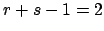. First, one with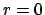and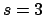:

> K<a> := NumberField(x^6+x+1);
> Signature(K);
0 3
> G, phi := UnitGroup(K);
> G;
Abelian Group isomorphic to Z/2 + Z + Z
Defined on 3 generators
Relations:
2*G.1 = 0
> u1 := K!phi(G.2); u1;
a
> u2 := K!phi(G.3); u2;
-2*a^5 - a^3 + a^2 + a
> Logs(u1);
[ 0.11877157353322375762475480482285510811783185904379239999998,
0.048643909752673399635150940533329986148342128393119899999997,
-0.16741548328589715725990574535618509426617398743691229999999 ]
> Logs(u2);
[ 1.6502294567845884711894772749682228152154948421589999999997,
-2.09638539134527779532491660083370951943382108902299999999997,
0.44615593456068932413543932586548670421832624686433469999994 ]

Notice that the log image of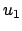is clearly not a real multiple of the log image of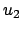(e.g., the scalar would have to be positive because of the first coefficient, but negative because of the second). This illustrates the fact that the log images ofandspan a two-dimensional space.

Next we compute a field with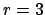and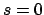. (A field withis called totally real''.)

> K<a> := NumberField(x^3 + x^2 - 5*x - 1);
> Signature(K);
3 0
> G, phi := UnitGroup(K);
> G;
Abelian Group isomorphic to Z/2 + Z + Z
Defined on 3 generators
Relations:
2*G.1 = 0
> u1 := K!phi(G.2); u1;
1/2*(a^2 + 2*a - 1)
> u2 := K!phi(G.3); u2;
a
> Logs(u1);
[ 1.16761574692758757159598251863681302946987760474899999999995,
-0.39284872458139826129179862583435951875841422643044369999996,
-0.7747670223461893103041838928024535107114633783181766999998 ]
> Logs(u2);
[ 0.6435429462288618773851817227686467257757954024463081999999,
-1.6402241503223171469101505551700850575583464226669999999999,
0.9966812040934552695249688324014383317825510202205498999998 ]


A family of fields with(totally complex) is the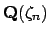. The degree ofover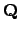is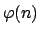and, so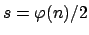(assuming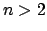).

> K := CyclotomicField(11); K;
Cyclotomic Field of order 11 and degree 10
> G, phi := UnitGroup(K);
> G;
Abelian Group isomorphic to Z/22 + Z + Z + Z + Z
Defined on 5 generators
Relations:
22*G.1 = 0
> u := K!phi(G.2); u;
zeta_11^9 + zeta_11^8 + zeta_11^7 + zeta_11^6 + zeta_11^5 +
zeta_11^3 + zeta_11^2 + zeta_11 + 1
> Logs(u);
[ -1.25656632417872848745322215929976803991663080388899999999969,
0.6517968940331400079717923884685099182823284402303273999999,
-0.18533004655986214094922163920197221556431542171819269999999,
0.5202849820300749393306985734118507551388955065272236999998,
0.26981449467537568109995283662137958205972227885009159999993 ]
> K!phi(G.3);
zeta_11^9 + zeta_11^7 + zeta_11^6 + zeta_11^5 + zeta_11^4 +
zeta_11^3 + zeta_11^2 + zeta_11 + 1
> K!phi(G.4);
zeta_11^9 + zeta_11^8 + zeta_11^7 + zeta_11^6 + zeta_11^5 +
zeta_11^4 + zeta_11^3 + zeta_11^2 + zeta_11
> K!phi(G.5);
zeta_11^9 + zeta_11^8 + zeta_11^7 + zeta_11^6 + zeta_11^5 +
zeta_11^4 + zeta_11^2 + zeta_11 + 1


How far can we go computing unit groups of cyclotomic fields directly with ?

> time G,phi := UnitGroup(CyclotomicField(13));
Time: 2.210
> time G,phi := UnitGroup(CyclotomicField(17));
Time: 8.600
> time G,phi := UnitGroup(CyclotomicField(23));
.... I waited over 10 minutes (usage of 300MB RAM) and gave up.


William Stein 2004-05-06